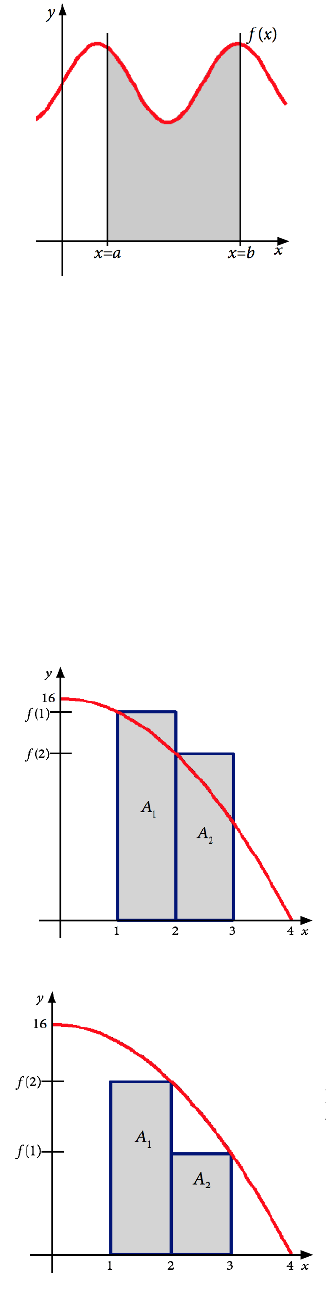Study Guides (400,000)
US (230,000)
NCSU (600)
MA (50)
MA 141 (40)
Final

# MA 141 Lecture 21: Areas and Riemann SumsExam

Department
Mathematics
Course Code
MA 141
Professor
Kevin Flores
Study Guide
Final

This preview shows pages 1-2. to view the full 6 pages of the document.
MA 141 Chapter 4
Section 4.1: Areas and Riemann Sums
To develop the notion of a deﬁnite integral, we will need a special notation for long sums.
This is called summation notation.
n
X
i=1
ai=a1+a2+. . . +an1+an
The capital Greek letter Σis the symbol for sum and the symbol airepresents the ith element
in the summation. The letter iis called the index of summation. This sum starts at i= 1
and goes up to n. Not all sums start at 1 and other letters could be used for the index of
summation.
Ex: Evaluate the sum
6
X
i=1
3i.
6
X
i=1
3i= 3(1) + 3(2) + 3(3) + 3(4) + 3(5) + 3(6)
= 3 + 6 + 9 + 12 + 15 + 18 = 63
Algebra of Summation: Let nbe a natural number, aiand bibe real numbers for ifrom
1 to n, and cR. Then
1)
n
X
i=1
(ai±bi) =
n
X
i=1
ai±
n
X
i=1
bi
2)
n
X
i=1
c·ai=c
n
X
i=1
ai
Ex: Evaluate the sum
4
X
j=1
(2j2+ 3).
4
X
j=1
(2j2+ 3) = 2
4
X
j=1
j2+
4
X
j=1
3
= 2[(1)2+ (2)2+ (3)2+ (4)2] + 3 + 3 + 3 + 3
= 2[1 + 4 + 9 + 16] + 12 = 2(30) + 12
= 72
1

Only pages 1-2 are available for preview. Some parts have been intentionally blurred.[ ]
Figure 1
Now suppose we wanted to ﬁnd the area of
a space bounded by a curve y=f(x), the
x-axis, and the lines x=aand x=b, as
in the image to the left.
There is no simple formula we can use. Instead, we will approximate the area.
The simplest shape we can use to approximate is a rectangle. We will slice the region into
rectangular strips and add up the areas. The thinner our strips, the more accurate the
estimation will be.
Ex: Let f(x) = 16 x2. Using two rectangles, estimate the area between the curve and the
x-axis on the interval [1,3].
If we use two rectangles, we want them to be the same width, so we will split the interval
into two - [1,2] and [2,3], both of width 1. How should we draw in the rectangles? We have
three basic choices:
Figure 3
im
h
f
3.
w
t
We could draw in rectangles using
the function value at the left-hand
endpoint of each interval. This is
called a left sum.
A1=f(1) ·1 = (16 12)·1 = 15
A2=f(2) ·1 = (16 22)·1 = 12
AL=A1+A2= 27
Figur
t
We could draw in rectangles using
the function value at the right-hand
endpoint of each interval. This is
called a right sum.
A1=f(2) ·1 = (16 22)·1 = 12
A2=f(3) ·1 = (16 32)·1 = 7
AR=A1+A2= 19